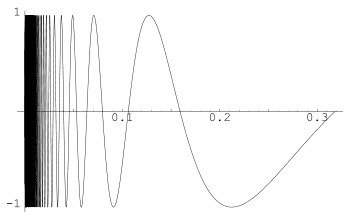# Question on Boundary Points

Question on Boundary Points!!

Determine all boundary points:

S={(x,y); 0 < x< 1 and y= sin(1/(1-x)}

I'm really confused! I dont understand how I can actually find all the points.. can anyone get me started??

The help is appreciated!

Office_Shredder
Staff Emeritus
Gold Member

S is going to be a curve, so it looks like it's asking you to find what's the boundary at x=0 and at x=1. At x=1 of course you're going to run into a snag because 1/(1-x) doesn't act so nice there, so start by finding the boundary point at x=0

Hurkyl
Staff Emeritus
Gold Member

Maybe start somewhere eaiser -- can you find any boundary points at all? Can you find all boundary points that lie in some easily-described subset of the plane?

Hey! Thats funny I have the same question in my practice questions too!
Anyone know how to do it? My prof gives no examples!

Hurkyl
Staff Emeritus
Gold Member

You could try starting with an easier problem -- can you find any boundary points at all? Can you find all boundary points that lie in some easily-described subset of the plane?

well sin(x) would probably be easier but how to find the actual points. if u take x=0 then sin (0)=0 and sin(1)=π/2

haha yah dont feel bad im pretty lost too!

Above ur taking the new function of y to be sin(x) right? .. this seems wrong lol no offence

k me and tara123 clearly dont know what were talking about can anyone help us???

Office_Shredder
Staff Emeritus
Gold Member

So for example, if you had S={(x,y)|y=x2+1 0<x<1} you can see that the boundary points of that curve are going to be exactly at (0,1) and (1,2). Now why is that. For any e>0, you can find a point on the curve S (x,y) such that |(x,y)-(0,1)|<e and also for any e>0, you can find a point on the curve (x,y) such that |(x,y)-(1,2)|<e. It's pretty easy here because y=x2+1 is a continuous function, so if you're told all the points x>0 are included, then the value at x=0 must be included.

So for the set S that you have, it's continuous away from x=1. So by the same process, we conclude that it has a boundary point at x=0. You should be able to find that point by yourself.

The tricky part is for x=1. It's not continuous there. What's the behavior of sin(1/(1-x)) as x approaches 1? What values of (1,y) might be part of the boundary?

HallsofIvy
Homework Helper

First, have you drawn a sketch of the set?
S={(x,y); 0 < x< 1 and y= sin(1/(1-x)} is essentially the graph of y= sin(1/(1-x)) for 0< x< 1. (One thing I would be inclined to do is let u= 1-s so this is y= sin(1/u) for 0< 1< u.) Look closely at what happens around u= 0 (x= 1).I found an image for when y=sin(1/u), but when you look at u=0, there is never a defined value for it as the function doesn't exist at u=0. I don't see how that would apply to our function.

yah so for x=0 y=sin(1)=pi
and for x=1 i just look at what the graph does as it approaches 0?

sorry i meant sin(1)=PI/2

but just one other thing, ive bin thinking and y=sin(1/1-x) o<x<1 0 isnt even contained in the set...so how is it a boundary point if 0 is strictly less than x???
Help!